International Journal of Energy and Power Engineering
Volume 5, Issue 1-1, February 2016, Pages: 53-56

Loss Calculation and Optimization Design of High Frequency Transformer

Lin Li*, Keke Liu, Xiaoying Zhang, Ning Zhang

Department of Electrical and Electronics Engineering, North China Electric Power University, Beijing, China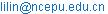(Lin Li)

Lin Li, Keke Liu, Xiaoying Zhang, Ning Zhang. Loss Calculation and Optimization Design of High Frequency Transformer. International Journal of Energy and Power Engineering. Special Issue: Numerical Analysis, Material Modeling and Validation for Magnetic Losses in Electromagnetic Devices. Vol. 5, No. 1-1, 2016, pp. 53-56. doi: 10.11648/j.ijepe.s.2016050101.18

Abstract: In this paper, the magnetization and loss properties are analyzed and compared of topical magnetic materials. The calculation methods are studied for the losses of the core and windings of the High Frequency Transformer (HFT). Based on the evaluation of the temperature increment, an optimization method is presented for the design of HFT. Finally, the losses of a test model of HFT is calculated and the results are compared with those tested.

Keywords: High Frequency Transformer, Magnetic Materials, Loss, Optimization

1. Introduction

In power electronic devices, such as DC/DC converter and solid state transformer, the operational frequencies of the transformers are chosen from several hundred Hz to several tens kHz in order to decrease their volumes and weights. This kind of transformers is called as High Frequency Transformer (HFT). The magnetization and loss mechanism of magnetic materials are related with the microscopic magnetization processes. In engineering application, the losses of soft magnetic material can usually be divided in three parts: eddy current loss, hysteresis loss and excess loss . The Steinmetz formula is widely used to calculate the loss of magnetic core of transformer . The losses of windings of high frequency transformer are related with the operation frequency, and the coefficient of alternative current resistance is used to reflect the skin effect and the proximity effect .

In this paper, the magnetization and loss properties are analyzed and compared of topical magnetic materials. The calculation methods of the losses of the core and windings of the HFT are analyzed. An optimization method is presented for the design of HFT. The losses of a test model of HFT is calculated and the results are compared with those tested.

2. Magnetization and Loss Properties of Topical Magnetic Materials

2.1. Silicon Steel Sheets

The superiorities of silicon steel sheets include: high saturation flux density, high stacking factor, high mechanical strength, good ductility, easy to cut, and low price, which let silicon steel sheets popularly used as the basic elements of lamination core of power transformer. But the eddy current and hysteresis losses of silicon steel sheets increase quickly with the increase of operation frequency, even if the sheets are ultrathin strips.

2.2. Amorphous Alloys

Amorphous alloys are also called as metallic glasses. When amorphous alloys are cooled from the liquid state and solidified as noncrystalline materials whose cooling rate is estimated to be in the range 105–106K/sec. The ribbons of amorphous alloys are usually a few millimeters wide, 25–35 nm thick, and meters to kilometers in length. The losses of amorphous alloys for unit volume are much lower than silicon steel sheets, especially in high frequencies. Therefore, amorphous alloys are usually chosen as the magnetic material for high frequency small capacity transformers and distribution power transformers. Maximum saturation magnetization is in the range 1.5–1.9 T, which is much larger than ferrites. Based on the design principle of transformer, rising the working flux density of the transformer core can decrease the turn number of the windings, and further the volume and weight of the high frequency transformer.

2.3. Nanocrystalline Alloys

A related class of nanocrystalline alloys is made by adding small amounts of Cu and Nb to an Fe–Si–B amorphous alloy. The most-studied composition is Fe74Si15B7Cu1Nb3. The Cu is believed to enhance nucleation of crystallites and the Nb to inhibit their growth. The saturation flux density of nanocrystalline alloys is high as amorphous alloys, and the high frequencies losses of nanocrystalline alloys for unit volume are lower than amorphous alloys. The price for unit mass of nanocrystalline alloys is so much higher than amorphous alloys that the material has not been applied in industry. In other hand, because the material is extremely brittle, it is difficult to manufacture magnetic core of nanocrystalline alloys with gaps.

2.4. Soft Ferrites

Ferrites have good properties of high frequencies losses. As a soft magnetic material, ferrites are widely used in small high frequency switching power supply, in which the operation frequency is from several kHz to several MHz. The saturation flux density of ferrites is lower as 0.2T so that the operation frequency chosen must be higher to make up the deficiency and lower the turn number of the windings of the transformer. Because ferrites are farinose, and unfavorable machining, the cores of ferrites are annular or closed UU shape without gaps.

2.5. Optimized Magnetic Material for High Frequency Transformer

The loss properties of silicon steel sheets, amorphous alloys, nanocrystalline alloys, and soft ferrites in 10 kHz and 50 kHz are shown in Fig.1. Loss properties and saturation flux densities of four magnetic core of silicon steel sheets, amorphous alloys, nanocrystalline alloys, and soft ferrites, are tested and listed in table 1. The saturation flux densities, losses for unit volume, machining properiesy and prices for unit mass are the main factors to choose magnetic material for high frequency transformers. From Fig.1 and table 1, it can be seen that the optimized magnetic materials are amorphous alloys or nanocrystalline alloys for high frequency transformer with several kHz operation frequency.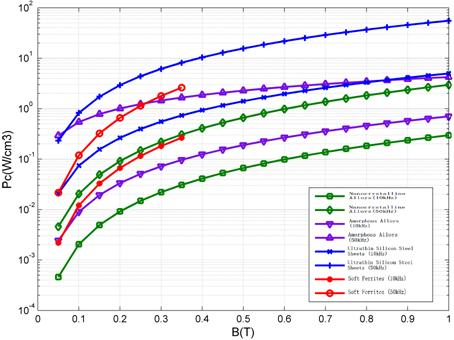Figure 1. Loss properties of ultrathin silicon steel sheets, amorphous alloys, nanocrystalline alloys, and soft ferrites.

Table 1. Loss properties and saturation flux densities of four magnetic cores.

 Magnetic material Saturation flux densities (T) Loss properties ([W/cm3]) Ferrites 0.35T Pc=0.1334B2.464[T]f1.422[kHz] amorphous alloys 1.2T Pc=0.0536B1.891[T]f 1.117[kHz] nanocrystalline alloys 1.3T Pc=0.0111 B2.161[T]f 1.428[kHz] ultrathin silicon steel sheets 1.5T Pc=0.1593B1.827[T]f 1.496[kHz]

3. Losses of High Frequency Transformer

3.1. Loss of Magnetic Core

Usually, losses of magnetic core can be divided in three parts: eddy current loss, hysteresis loss and excess loss. The losses of magnetic core per unit mass can be calculated by :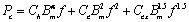(1)

where Ch, Ce, and Cex are the coefficients of eddy current loss, hysteresis loss and excess loss, respectively. f is the operation frequency of high frequency transformer, and Bm is the maximum magnetic flux density of the core. a is an undetermined index, which is related with specified magnetic material. Another reduced core loss calculation formula is as follows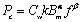(2)

where Cw is the coefficients related with the waveform of the magnetic flux density, 1 for sinusoidal wave, p/4 for square wave, and 2/3 for triangle wave. k, a and b are undetermined indexes related with specified magnetic material. (2) is also called Steinmetz formula.

3.2. Losses of the Windings

The loss of winding of transformer can be calculated by: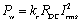(3)

where RDC is the direct current resistance of the winding, and Irms is the root mean square current. kr (=RAC/RDC) is the coefficient of alternative current resistance, which can be expressed as follows :(4)

where m is the number of layers of the winding, and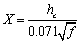, in which hc is the thickness of the conductor wires.

3.3. A Model of High Frequency Transformer

A model of high frequency transformer is made and shown in Fig.2, whose basic parameters are listed in table 2. The zero-load losses of the transformer for different frequencies are tested that can be used to represented the losses of core. The calculation formula of loss of core is obtained by means of the tested data, and it is as follows: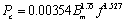(5)

Table 2. Basic parameters of the model of high frequency transformer.

 Magnetic material Saturation flux densities (T) Voltages of windings (V) Frequency (Hz) Capacity (kVA) 0.3mm silicon steel sheets 0.7 560/160 400 1.5

The calculated and test losses of core are listed in table 3. The calculated and test losses of windings are listed in table 4. It can be seen from table 3-4 that the errors are small between the calculated and the tested losses of the core and windings.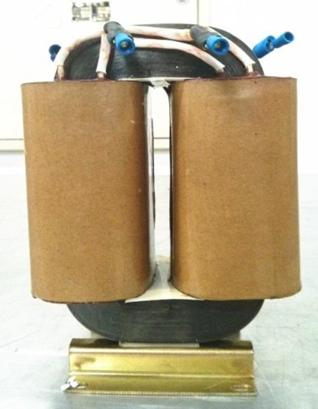Figure 2. Model of high frequency transformer.

Table 3. Calculated and test losses of core.

 f (Hz) Calculated Pc (W) Tested Pc (W) Error(％) 50 0.740 0.7 5.71 60 0.935 0.9 3.89 100 1.900 2.0 -5.00 200 5.304 5.5 -3.56 300 10.470 10.35 1.15

Table 4. Calculated and test losses of windings.

 f (Hz) Calculated Pw (W) Tested Pw (W) Error(％) 50 32.5 31.05 4.67 60 32.5 31.05 4.67 100 32.8 31.25 4.96 200 33.3 32.68 1.90 300 35.3 35.10 0.57 400 38.2 38.13 0.18

4. Optimization Design of High Frequency Transformer

One of the optimization design objects of high frequency transformer is to determine the optimum magnetic flux density Bopt corresponding to the minimum total loss Pt of the transformer and the determined operation frequency. Let the derivative of the total loss Pt to the magnetic flux density B equal to zero, that is,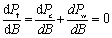(6)

We can obtained the optimum magnetic flux density Bopt as: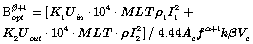(7)

where k, a and b are the indexes related with specified magnetic material and the same as that in (2). Uin and Uout are the voltages of the primary winding and the secondary winding, respectively. I1 and I2 are the currents of the primary winding and the secondary winding, respectively. Vc and Ac are the volume and across area of the magnetic core, respectively. K1 and K2 are the coefficient of alternative current resistance of the primary winding and the secondary winding, respectively. MLT is the average turn length of the windings and ρ is the resistivity of conductor of of the windings.

For one kind of amorphous alloys, the calculation formula of the loss of magnetic core is: Pc(W/cm3)=0.0306B1.74f(kHz)1.51. The optimization design results of a 10 kVA high frequency transformer are shown in table 5. For one kind of nanocrystalline alloys, the calculation formula of the loss of magnetic core is: Pc(W/cm3)=0.008B1.982f(kHz)1.621. The optimization design results of a 10 kVA high frequency transformer are shown in table 6.

5. Conclusions

Based on the study of the paper, we obtained the following conclusions:

(1)  For the large capacity high frequency, the optimization magnetic materials are amorphous alloys and nanocrystalline alloys which have better magnetization and loss properties.

(2)  The Steinmetz formula can be used to calculate the loss of core, and the method of coefficient of alternative current resistance to the losses of windings of HFT.

Table 5. Optimization design results of a 10 kVA amorphous alloys high frequency transformer.

 f(kHz) Bop(T) Vc(cm3) S/Vc Pc(W) Pcu(W) Pt(W) 10 0.2939 259.9911 39.84205 30.5711 53.5436 84.1146 20 0.1574 241.6788 41.37723 27.3037 47.7399 75.0436 30 0.1106 229.5788 43.55803 25.8983 45.3952 71.2935 40 0.0951 203.5538 49.12706 26.3105 46.1546 72.4651 50 0.077 192.6796 51.89963 27.059 44.5896 71.6486 80 0.0472 232.6637 42.98049 26.1927 45.7791 71.9718 100 0.0375 250.1911 39.96945 26.4792 46.2359 73.705

Table 6. Optimization design results of a 10 kVA nanocrystalline alloys high frequency transformer.

 f(kHz) Bop(T) Vc(cm3) S/Vc Pc(W) Pcu(W) Pt(W) 10 0.5633 129 77.51938 13.8365 27.6104 41.4469 20 0.3217 113.9947 87.7233 12.3767 24.6451 37.0218 30 0.2349 105.8522 94.4713 11.8885 23.5591 35.4476 50 0.1486 104.5664 95.6330 10.8533 21.8397 32.693 80 0.1011 104.5664 95.6330 10.8344 21.4794 32.3138 100 0.0799 112.2372 89.0970 10.4679 20.9212 31.3891

References

1. Boglietti ACavagnino ALazzari Met al. Predicting iron losses in soft magnetic materials with arbitrary voltage supply: an engineering approach [J]. IEEE Trans. Magn., 39(2): pp.981-989, March 2003.
2. Bertotti G. General properties of power losses in soft ferromagnetic materials[J].IEEE Trans. Magn., 24(1): pp621-630, January, 1988.
3. P.L.Dowell. Effect of eddy currents in transformer windings[J]. Proc. Inst. Elect. Eng., 113, pp1387-1394, 1996.
4. I. D. Mayergoyz, G. Friedman, and C. Salling, "Comparison of the classical and generalized Preisach hysteresis models with experiments,"IEEE Trans. Magn., vol. 25, no. 5, pp. 3925–3927, Sep. 1989.
5. E. Barbisio, F. Fiorillo, and C. Ragusa, "Predicting loss in magnetic steels under arbitrary induction waveform and with minor hysteresis loops," IEEE Trans. Magn., vol. 40, no. 4, pp. 1810–1819, Jul. 2004.

 Contents 1. 2. 2.1. 2.2. 2.3. 2.4. 2.5. 3. 3.1. 3.2. 3.3. 4. 5.
Article ToolsAbstractPDF(2714K)